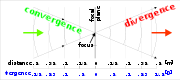xVergence (optics)EncyclopediaThe vergence of a light bundle
Ray (optics)
In optics, a ray is an idealized narrow beam of light. Rays are used to model the propagation of light through an optical system, by dividing the real light field up into discrete rays that can be computationally propagated through the system by the techniques of ray tracing. This allows even very...

in optics
Optics
Optics is the branch of physics which involves the behavior and properties of light, including its interactions with matter and the construction of instruments that use or detect it. Optics usually describes the behavior of visible, ultraviolet, and infrared light...

is the reciprocal of the distance between the point of focus
Focus (optics)
In geometrical optics, a focus, also called an image point, is the point where light rays originating from a point on the object converge. Although the focus is conceptually a point, physically the focus has a spatial extent, called the blur circle. This non-ideal focusing may be caused by...

and a reference plane. It is measured in dioptre
Dioptre
A dioptre, or diopter, is a unit of measurement of the optical power of a lens or curved mirror, which is equal to the reciprocal of the focal length measured in metres . It is thus a unit of reciprocal length. For example, a 3-dioptre lens brings parallel rays of light to focus at metre...

s (1 D = 1 m−1). Vergence can also be understood as a measure of the curvature
Curvature
In mathematics, curvature refers to any of a number of loosely related concepts in different areas of geometry. Intuitively, curvature is the amount by which a geometric object deviates from being flat, or straight in the case of a line, but this is defined in different ways depending on the context...

of the optical wavefront
Wavefront
In physics, a wavefront is the locus of points having the same phase. Since infrared, optical, x-ray and gamma-ray frequencies are so high, the temporal component of electromagnetic waves is usually ignored at these wavelengths, and it is only the phase of the spatial oscillation that is described...

. However, it is only fully valid in geometrical optics
Geometrical optics
Geometrical optics, or ray optics, describes light propagation in terms of "rays". The "ray" in geometric optics is an abstraction, or "instrument", which can be used to approximately model how light will propagate. Light rays are defined to propagate in a rectilinear path as far as they travel in...

, not in Gaussian beam
Gaussian beam
In optics, a Gaussian beam is a beam of electromagnetic radiation whose transverse electric field and intensity distributions are well approximated by Gaussian functions. Many lasers emit beams that approximate a Gaussian profile, in which case the laser is said to be operating on the fundamental...

optics or in wave optics where the wavefront
Wavefront
In physics, a wavefront is the locus of points having the same phase. Since infrared, optical, x-ray and gamma-ray frequencies are so high, the temporal component of electromagnetic waves is usually ignored at these wavelengths, and it is only the phase of the spatial oscillation that is described...

at the focus is wavelength
Wavelength
In physics, the wavelength of a sinusoidal wave is the spatial period of the wave—the distance over which the wave's shape repeats.It is usually determined by considering the distance between consecutive corresponding points of the same phase, such as crests, troughs, or zero crossings, and is a...

dependent and the curvature is not proportional to the distance from the focus.

Convergence describes the situation when the light rays are approaching each other and its values are positive.
Divergence is measured as negative dioptres and means that the beams are originating from a point.

• Optical power
Optical power
Optical power is the degree to which a lens, mirror, or other optical system converges or diverges light. It is equal to the reciprocal of the focal length of the device. The dioptre is the most common unit of measurement of optical power...

• Optical resolution
Optical resolution
Optical resolution describes the ability of an imaging system to resolve detail in the object that is being imaged.An imaging system may have many individual components including a lens and recording and display components...

• Cardinal point (optics)
Cardinal point (optics)
In Gaussian optics, the cardinal points consist of three pairs of points located on the optical axis of an ideal, rotationally symmetric, focal, optical system...

• Radius of curvature (optics)
Radius of curvature (optics)
Radius of curvature has specific meaning and sign convention in optical design. A spherical lens or mirror surface has a center of curvature located in either along or decentered from the system local optical axis. The vertex of the lens surface is located on the local optical axis...